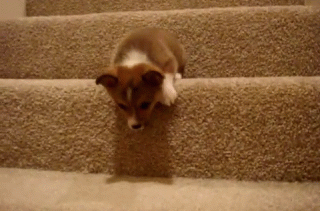IntroductionIntroduction# Dilations on a Graph

INTRO
A dilation is a transformation that changes the size of an image without changing its shape or proportions.
It's like how when Ant-Man gets bigger or smaller, each body part changes size by the same amount, so he looks the same - just bigger or smaller than before. Dilating shapes is similar 👯‍♀️.When we dilate shapes on a grid, we need to know the center of dilation and the scale factor.
To help us understand these terms, let’s imagine we have a movable screen and a fixed projector that can’t be moved:### Closer

✨ Drag to move the projection ✨

### Farther

The center of dilation is like the fixed projector: it’s a fixed point from which the image is drawn. The scale factor determines how much smaller or larger the dilated image will be.
If the scale factor is , we can imagine keeping the distance between the projector and the screen the same, which will make the new image the same as the original.
Check out our
Calculator
or explore our
Lesson
and
Practice

CALCULATOR

KEY STEPS

## How to Perform Dilations

### Step 1. Identify the center of dilation.

Imagine this as the fixed location of the projector.

### Step 2. Identify the original points of the polygon.

Imagine this as the original image before the screen is moved.

### Step 3. Identify the scale factor .

If the scale factor is , we can imagine keeping the distance between the projector and the screen the same, which will make the new image the same as the original.

### Step 4. Multiply each original point of the polygon by the scale factor to get the new points.

 Original Point Scale Factor New Point

### Step 5. Plot the new points and connect the dots to get your dilated shape.

LESSON
Dilations Centered at the Origin

## Dilations Centered at the Origin

When a dilation is centered at the origin, it means that the dilation is projected from the point .

In order to find the new points of a dilation centered at the origin, all we need to do is multiply the and coordinates of the original points by the scale factor.
Once we have the new points, we can plot them on the graph and connect the dots to reveal the new image. 🤩
Let’s walk through a couple examples!
PRACTICE
Dilations Centered at the Origin

## Practice: Dilations Centered at the Origin

Question 1 of 3: Dilate this figure by a scale factor of with a center of dilation at the origin.

### Step 1. Identify the center of dilation.

Imagine this as the fixed location of the projector.

LESSON
Dilations NOT Centered at the Origin

## Dilations NOT Centered at the Origin

Dilations that are not centered at the origin are a little trickier, but still 100% doable if we take it one step at a time.Let’s walk through a couple examples using our projector-screen idea!
PRACTICE
Dilations NOT Centered at the Origin

## Practice: Dilations NOT Centered at the Origin

Question 1 of 3: Dilate this figure by a scale factor of with a center of dilation at .

### Step 1. Identify the center of dilation.

Imagine this as the fixed location of the projector.

CONCLUSION
Incredible job, look at you go! Thanks for checking out this lesson ☺️🙏. Where to next?Leave Feedback